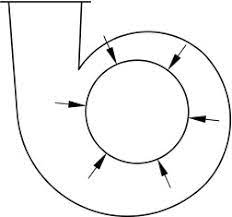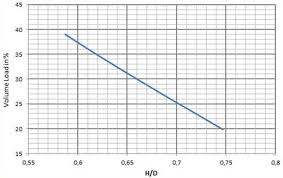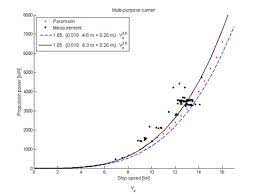## How to Calculate and Solve for Shaft Diameter | Ball Mill SizingThe image above represents shaft diameter.

To compute for shaft diameter, two essential parameter are needed and these parameters are Shaft Power (P) and 75% of Critical Speed (N).

The formula for calculating shaft diameter:

D = ³√(1.33 x 106 x P/N)

Where:

D = Shaft Diameter
P = Shaft Power
N = 75% of Critical Speed

Let’s solve an example;
Find the shaft diameter when the shaft power is 28 and the 75% of critical speed is 7.

This implies that;

P = Shaft Power = 28
N = 75% of Critical Speed = 7

D = ³√(1.33 x 106 x P/N)
D = ³√(1.33 x 106 x 28/7)
D = ³√(37240000/7)
D = ³√(5320000)
D = 174.57

Therefore, the shaft diameter is 174.57 m.

Calculating the Shaft Power when the Shaft Diameter and the 75% of Critical Speed is Given.

P = D3 x N / 1.33 x 106

Where;

P = Shaft Power
D = Shaft Diameter
N = 75% of Critical Speed

Let’s solve an example;
Find the shaft power when the shaft diameter is 3 and the 75% of critical speed is 10.

This implies that;

D = Shaft Diameter = 3
N = 75% of Critical Speed = 10

P = D3 x N / 1.33 x 106
P = 33 x 10 / 1.33 x 106
P = 9 x 10 / 1.33 x 106
P = 90 / 140.98
P = 0.64

Therefore, the shaft power is 0.64 m/s.

## How to Calculate and Solve for Shaft Power | Ball Mill LengthThe image above represents shaft power | ball mill length.

To compute for shaft power | ball mill length, six essential parameters are needed and these parameters are Value of C, Volume Load Percentage (J), % Critical Speed (Vcr), Bulk Density (s.g), Mill Length (L) and Mill Internal Diameter (D).

The formula for calculating shaft power | ball mill length:

P = 7.33 x C X J X Vcr x (1 – 0.937) x [1 – 0.1/29 – 10Vcr] x s.g. x L x D2.3

Where:

P = Shaft Power | Ball Mill Length
Vcr = % Critical Speed
sg = Bulk Density
L = Mill Length
D = Mill Internal Diameter

Let’s solve an example;
Find the shaft power | ball mill length when the volume load percentage is 8, the %critical speed is 10, the bulk density is 3. the mill length is 14 and the mill internal diameter is 16.

This implies that;

J = Volume Load Percentage = 8
Vcr = % Critical Speed = 10
sg = Bulk Density = 3
L = Mill Length = 14
D = Mill Internal Diameter = 16

P = 7.33 x C X J X Vcr x (1 – 0.937) x [1 – 0.1/29 – 10Vcr] x s.g. x L x D2.3
P = 7.33 x 1 x 8 x 10 x (1 – 0.937) x [1 – 0.1/29 – 10(10)] x 3 x 14 x 162.3
P = 36.94 x [1 – 0.1/2-91] x 3 x 14 x 588.133
P = 36.94 x [1 – 0.1/4.038e-28] x 24701.609
P = 36.94 x [1 – 2.47e+26] x 24701.609
P = 36.94 x -2.475e+26 x 24701.609
P = -2.259

Therefore, the shaft power | ball mill length is – 2.259 W.

## How to Calculate and Solve for Shaft Power | Ball Mill SizingThe image above represents shaft power.

To compute for shaft power, two essential parameters are needed and these parameters are Mill Capacity (Q) and Specific Power of Mill (E).

The formula for calculating shaft power:

P = QE

Where:

P = Shaft Power
Q = Mill Capacity
E = Specific Power of Mill

Let’s solve an example;
Find the shaft power when the mill capacity is 20 and the specific power of mill is 24.

This implies that;

Q = Mill Capacity = 20
E = Specific Power of Mill = 24

P = QE
P = (20)(24)
P = 480

Therefore, the shaft power is 480 W.

Calculating for the Mill Capacity when the Shaft Power and the Specific Power of Mill is Given.

Q = P / E

Where;

Q = Mill Capacity
P = Shaft Power
E = Specific Power of Mill

Let’s solve an example;
Find the mill capacity when the shaft power is 24 and the specific power of mill is 6.

This implies that;

P = Shaft Power = 24
E = Specific Power of Mill = 6

Q = P / E
Q = 24 / 6
Q = 4

Therefore, the mill capacity is 4.
Continue reading How to Calculate and Solve for Shaft Power | Ball Mill Sizing